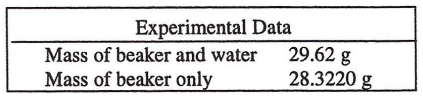# Problem: What is the correctly reported mass of water based on this data? (A) 1.2980 g(B) 1.298 g(C) 1.30 g(D) 1.3 g

###### FREE Expert Solution
79% (305 ratings)
###### Problem Details

What is the correctly reported mass of water based on this data?

(A) 1.2980 g

(B) 1.298 g

(C) 1.30 g

(D) 1.3 gWhat scientific concept do you need to know in order to solve this problem?

Our tutors have indicated that to solve this problem you will need to apply the Significant Figures: In Calculations concept. You can view video lessons to learn Significant Figures: In Calculations. Or if you need more Significant Figures: In Calculations practice, you can also practice Significant Figures: In Calculations practice problems.

What is the difficulty of this problem?

Our tutors rated the difficulty ofWhat is the correctly reported mass of water based on this d...as low difficulty.

How long does this problem take to solve?

Our expert Chemistry tutor, Dasha took 1 minute and 50 seconds to solve this problem. You can follow their steps in the video explanation above.

What professor is this problem relevant for?

Based on our data, we think this problem is relevant for Professor Sempertegui's class at FAU.